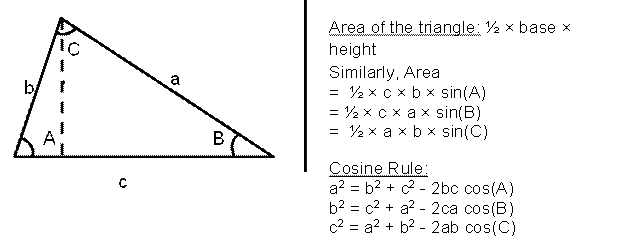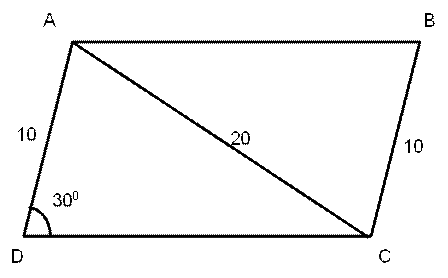# CAT 2021 Question Paper | Quant Slot 3

###### CAT Previous Year Paper | CAT Quant Questions | Question 11

CAT 2021 Quant was dominated by Arithmetic followed by Algebra. In Arithmetic, the questions were dominated by topics like Speed-time-distance, Mixture and Alligations. This year, there was a surprise. The questions from Geometry were relatively on the lower side as compared to the previous years. There were 8 TITA Qs this year. Overall this section was at a medium level of difficulty.

Question 11 : Let ABCD be a parallelogram. The lengths of the side AD and the diagonal AC are 10 cm and 20 cm, respectively. If the angle ∠ADC is equal to 30° then the area of the parallelogram, in sq. cm, is

1. $$frac{25$$sqrt{3}+$sqrt{15}$}{2}$ 2. $25$$sqrt{5}+$sqrt{15}$$ 3. $$frac{25$$sqrt{5}+\sqrt{15}$}{2}$ 4. $25$$sqrt{3}+$sqrt{15}$$ ## Best CAT Online Coaching Try upto 40 hours for free Learn from the best! #### 2IIM : Best Online CAT Coaching. ### Video Explanation ## Best CAT Coaching in Chennai #### CAT Coaching in Chennai - CAT 2022Limited Seats Available - Register Now! ### Explanatory Answer Revising the Cosine rule and the area of the triangle using the Sine rule…We draw the described parallelogram ABCD.Applying the cosine rule in triangle ADC, 202 = 102 + DC2 - 2$10)(DC) cos(30)
400 = 100 + DC2 - 2(10)(DC)($$frac { $sqrt { 3 } } { 2 }$$ 300 = DC2 -$10)(DC)($$sqrt { 3 }$) DC2 -$10)($$sqrt { 3 }$)$DC) - 300 = 0
DC = $$frac {$ $sqrt { 3 } )$ 10 ) $pm $sqrt {$ $sqrt { 3 } 10$ ^ { 2 } - 4$ 1 ) ( - 300 ) } } { 2 }$
DC is the length of one of the sides of the parallelogram, hence it can’t be negative.
DC = $$frac {$ $sqrt { 3 } )$ 10 ) + $sqrt {$ 300 ) 5 } } { 2 }$
DC = $$frac { $sqrt {$ 300$ 5 } + ( $sqrt { 3 } )$ 10 ) } { 2 }$
DC = $$frac { 10 $sqrt { 15 } + 10 \sqrt { 3 } } { 2 }$ DC = 5$$$sqrt { 15 } + \sqrt { 3 }$$ The are of the triangle ADC = ½ × DC × 10 × sin$300) (Applying the sine rule)
Area(ADC) = ½ × DC × 10 × sin(300)
Area(ADC) = $$frac { 10 $mathrm { DC } } { 4 }$= $\frac { 5 D C } { 2 }$ Area of the parallelogram ABCD = 2 × Area$ADC$
Area of the parallelogram ABCD = 2 × $$frac { 5 D C } { 2 }$ Area of the parallelogram ABCD = 5$DC)
Area of the parallelogram ABCD = 5 ( 5($$sqrt { 15 } + $sqrt { 3 }$$) Area of the parallelogram ABCD = 25$$$sqrt { 15 } + $sqrt { 3 }$$ ##### The answer is '$25$$sqrt{3}+$sqrt{15}$$' Choice D is the correct answer. ###### Prepare for CAT 2023 with 2IIM's Daily Preparation Schedule ###### Know all about CAT Exam Syllabus and what to expect in CAT ###### Best CAT Online Coaching Try upto 40 hours for free Learn from the best! ###### Already have an Account? ###### CAT Coaching in ChennaiCAT 2023 Classroom Batches Starting Now! @Gopalapuram ###### Best CAT Coaching in Chennai Introductory offer of 5000/- Attend a Demo Class ###### Best Indore IPM & Rohtak IPM CoachingSignup and sample 9 full classes for free. Register now! ##### Where is 2IIM located? 2IIM Online CAT Coaching A Fermat Education Initiative, 58/16, Indira Gandhi Street, Kaveri Rangan Nagar, Saligramam, Chennai 600 093 ##### How to reach 2IIM? Mobile:$91) 99626 48484 / 94459 38484
WhatsApp: WhatsApp Now
Email: info@2iim.com